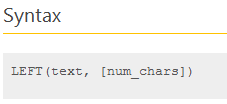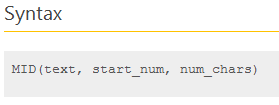# Formula Forensic No 019. Converting uneven Text Strings to Time

Share

Last week over at the Chandoo.org Forums, Birko asked a question about How to Import some Numbers as Times.

I have imported some data that comes in as a number that I need to convert to h:mm. The data string will be either 1,3,4,5,6 integers long and looks like this…eg

Imported         Need to equal this
Number           h:mm
0                      0:00
100                  0:01
1000                0:10
10900              1:09
235900            23:59

Can someone please provide a smart formula to convert this (assume data is in cell A1).

Today in Formula Forensics we will look at how this problem was solved, and the solution which may surprise you.

## Importing Numbers as Times.

When I first saw this data I start by looking at patterns.

Working backwards through the list

I can see that 235900 is 23 Hrs, 59 Min and 0 second

I can see that 10900 is 1 Hr, 9 Min and 0 second

I can see that 1000 is 0 Hrs, 10 Min and 0 second

I can see that 100 is 0 Hr, 1 Min and 0 second

I can see that 0 is 0 Hr, 0 Min and 0 second

I then start to think about how to extract the Hours, Minutes and seconds independently from the Text using a series of Left, Right and Mid functions, and quickly realised that due to the varying lengths of the strings, That they will end up being complex formulas as I will need to allow for each string length.

What if I pad the strings with leading 0’s and then extract them.

That is possible, but as a single formula it will be long and cumbersome as the padding has to occur a number of times for each Hour, Minute and Second as part of the Time() function.

So padding may work but is cumbersome, then a bright light moment

And I quickly posted the following formula:

``=(LEFT(TEXT(A1,"000000"),2)/24)+(MID(TEXT(A1,"000000"),3,2)/1440)+(RIGHT(TEXT(A1,"000000"),2)/(24*3600))``

As Time is just a number between 0 = midnight and 0.999999 = 11:59:59 pm, I can extract the Hours, Minutes and seconds separately and then simply add them together to get the actual time

I Can use the Text function to display the Strings in a consistent format that allows me to use the Left, Mid and Right functions to retrieve the Hours minutes and Seconds from the appropriate places.

Lets work through this formula section by section and see what is going on.

### Hours

`The Hours component of the formula is`

`=``(LEFT(TEXT(A1,"000000"),2)/24)``+(MID(TEXT(A1,"000000"),3,2)/1440)+(RIGHT(TEXT(A1,"000000"),2)/(24*3600))`

`=(LEFT(TEXT(A1,"000000"),2)/24)`

Working from the middle out, this formula takes the value in A1 and displays it as a Number with the format “000000”

So using our data

235900 will convert to 235900

10900 will convert to 010900

1000 will convert to 001000

We can now use a Left() function to extract the hours from the first 2 characters of the converted stringUsing our examples:

Left(235900,2) = 23

Left(010900,2) = 01

Left(001000,2) = 00

To convert hours to a Time we simply divide by 24

### Minutes

`The Minutes component of the formula is`

`=(LEFT(TEXT(A1,"000000"),2)/24)+(``MID(TEXT(A1,"000000"),3,2)/1440``)+(RIGHT(TEXT(A1,"000000"),2)/(24*3600))`

`=MID(TEXT(A1,"000000"),3,2)/1440`

Once again, Working from the middle out, this formula takes the value in A1 and displays it as a Number with the format “000000”

So using our data

235900 will convert to 235900

10900 will convert to 010900

1000 will convert to 001000

We can now use a Mid() function to extract the minutes from the middle 2 characters of the converted stringMid(235900,3,2) = 59

Mid(010900,2) = 09

Mid(001000,2) = 10

To convert Minutes to a Time we simply divide by 1440 (1440 is how many minutes are in a day = 24 * 60)

### Seconds

`The Seconds component of the formula is`

``=(LEFT(TEXT(A1,"000000"),2)/24)+(MID(TEXT(A1,"000000"),3,2)/1440)+(``RIGHT(TEXT(A1,"000000"),2)/(24*3600)``)``
``=RIGHT(TEXT(A1,"000000"),2)/(24*3600)``)``

Once again, Working from the middle out, this formula takes the value in A1 and displays it as a Number with the format “000000”So using our data

235900 will convert to 235900

10900 will convert to 010900

1000 will convert to 001000

We can now use a Right() function to extract the minutes from the middle 2 characters of the converted string

Right(235900,3,2) = 00

Right(010900,2) = 00

Right(001000,2) = 00

To convert Seconds to a Time we simply divide by 86400 (86,400 is how many seconds are in a day = 24 * 60 * 60)

### Total Time

To get the total Time we simply add the Hour, Minutes and Seconds together

`=``(LEFT(TEXT(A1,"000000"),2)/24)``+``(MID(TEXT(A1,"000000"),3,2)/1440)``+``(RIGHT(TEXT(A1,"000000"),2)/(24*3600)``)`

## Formula Forensics “The Series”

Formula Forensic Series

## Formula Forensics Needs Your Help

I urgently need more ideas for future Formula Forensics posts and so I need your help.

If you have a neat formula that you would like to share and explain, try putting pen to paper and draft up a Post like above or;

If you have a formula that you would like explained but don’t want to write a post also send it to Chandoo or Hui.

### Get FREE Excel + Power BI Tips

Simple, fun and useful emails, once per week.

Learn & be awesome.

### Welcome to Chandoo.org

Thank you so much for visiting. My aim is to make you awesome in Excel & Power BI. I do this by sharing videos, tips, examples and downloads on this website. There are more than 1,000 pages with all things Excel, Power BI, Dashboards & VBA here. Go ahead and spend few minutes to be AWESOME.

Read my storyFREE Excel tips book

I will be presenting at two events in London in April 2020. Come & join me.

Chandoo is an awesome teacher
5/5

– Jason

Still on fence about Power BI? In this getting started guide, learn what is Power BI, how to get it and how to create your first report from scratch.

## Related Tips

### 22 Responses to “Formula Forensic No 019. Converting uneven Text Strings to Time”

1.Joe Carsto says:

Why not let the TIME function take care of the math:
=TIME(LEFT(TEXT(A1,"000000"),2),MID(TEXT(A1,"000000"),3,2),RIGHT(TEXT(A1,"000000"),2))

•Ben Niebuhr says:

I was going to point out the same thing, except to note that useing the time function and doing the divide method are not interchangeable.

I have spent hours investigating a spreadsheet working with a couple of years worth of hourly data, and found that the reason things weren't working is because the rounding on the divide method is only close to the correct time values. In order to have it work for comparisons, (like sub-totaling by time value, or pivoting) you MUST use the TIME function.

Great use of the TEXT function, Hui. I will be using this concept for sure.

2.Elias says:

Why not just.

=TEXT(A1,"00\:00\:00")*1

Regards

•Joe Carsto says:

Elegant!

•Manick says:

Hi Elias,

I tried to use your formula. But, it doesn't seem to work for me. I am getting an error message "The formula you typed contains an error". It seems I have the problem in using \: in the format. How can I overcome this?

Thanks

•Greg G says:

Manick, it isn't the /: that causes the problem. If you copy/paste it, you're getting “'s instead of the actual quotation marks that Excel uses. Change the quotation marks by deleting from the pasted formula and retype them.

•modeste says:

Hi Manick...
use this alternate formula :
=1*TEXT(A1,"00"":""00"":""00")

note twice double quote each side of :

3.Elias says:

@Manick,

Did you copy the formula and pasted in Excel or did you typed? Also, do you use , or ; as separator of arguments?

Regards

•Joe Carsto says:

@Elias: I had no problem using your formula, in fact, I have used your method to convert a number such as 20120419 to an Excel date using =TEXT(A1,"0000\/00\/00")*1. Thanks for posting.

•Elias says:

@Joe: For date convertion you can use this as well.

=TEXT(A1,"00-00-00")*1

Regards

•Joe Carsto says:

Sweet! It appears this also works with =TEXT(A1,"0-00-00")*1. I come from the old days when you counted every byte. I also like to try an make formulas as small as possible for the fun of it 🙂

4.Haseen says:

Elias's suggestion is the simplest, but here is yet another way with TIME and MOD functions...

=TIME(MOD(A2/10000,100),MOD(A2/100,100),MOD(A2,100))

5.Rick Rothstein (MVP - Excel) says:

Since the seconds appear to always be 0, why not simply the input to minutes and above and save yourself the trouble of typing those zeroes...

0 => 0:00
1 => 1:00
10 => 10:00
100 => 1:00:00
etc.

Then just use this formula...

=TEXT(A1,"0\:00\:")*1

•Elias says:

@ Rick, the numbers to convert are no typed, they are imported. Then your formula will return the wrong result.

Regards.

6.Rick Rothstein (MVP - Excel) says:

Hmm! My formula lost some backslash-zero combinations (two of them to be exact). The formula was supposed to be this...

=TEXT(A1,"0\:00\:\zero\zero")*1

where the words "zero" should actually be the number 0. Another way to write the formula is this...

=TEXT(A1,"0\:00\:""00""")*1

7.Rajagopal says:

Hi Master,
While writing the formulae you have considered only upto "seconds factor" . I think you should take the centi-seconds factor also to achieve best results. Please look into it and rectify the problem...?

For Example.
In horse racing timings are noted in minute, seconds and centi-seconds, like if a horse finished in 70 seconds over a scurry of 1200 metres, is noted as 1.10 min. Nowadays it is noted in centi-seconds everywhere, like 70.00 if you want to convert it to centi seconds (should multiply by 100) = 7000 centi seconds. If you put this figure into your formula as a general number (7000) it will return as 1:10:00. As per your formula, it should be taken as 1 hour 10 seconds 0 minutes. However for a racing enthusiast like me it can be taken as 1 minute 10 seconds also.

Just look what happens if we race goers use this figure as 7000 centi seconds in your formulae, it will correctly show as 1 minute 10 seconds(?) Suppose a horse finishing over a 1200m in 70.60 seconds or in racing terms written as 1.10.60 mins, where 1 minute 10 seconds, & 60 centi-seconds can be counted as 7060, if you put this figure in the formula it will return as 1 minute 11 seconds, that is correct.

My point is if you can incorporate Centi Seconds in the formulae, it would be of great help to us also.

Thanks and regards.
Rajagopal (Mumbai)

8.Vishy says:

Awesome techniques !

I tried with 235960 just to see if it will fail but this is great.

9.CMC says:

Although a little longer, this too work:

=CHOOSE(LEN(A2);A2/(24*3600);A2/(24*3600);LEFT(A2;1)/(24*60) + RIGHT(A2;2)/(24*3600);LEFT(A2;2)/(24*60) + RIGHT(A2;2)/(24*3600);LEFT(A2;1)/24 + MID(A2;2;2)/(24*60) + RIGHT(A2;2)/(24*3600);LEFT(A2;2)/24 + MID(A2;3;2)/(24*60) + RIGHT(A2;2)/(24*3600))

10.Android Rooted Apps says:

Converting uneven Text Strings to Time I have imported some data that comes in as a number that I need to convert to h:mm.

11.Sudhir Gawade says:

Just come across this while googling

find interesting challenge and come up with this

=TEXT(TEXT(SUBSTITUTE(A1,RIGHT(A1,1),""),"000000"),"00\:00\:00")

12.Renee Keel says:

I need to convert a string of numbers representing average minutes, to reflect correct time values. For example, the numbers below currently represent 5.79 minutes, 15.82 minutes, etc.

I need to convert these values to their correct corresponding value within time parameters. So 5.79 would be something close to 5 minutes and 45 seconds.

5.79
15.82
3.92
12.40
6.70
3.62

I know there has to be a way to compute this in Excel, it can do anything, I believe!

Thank you for any and all assistance~

•Chandoo says:

@Renee... You can use a formula like this. Assuming A1 has the minutes.seconds,

=INT(A1) + MOD(A1, 1)*0.6

If you want to see it in 5 minutes 45 seconds format, use

=INT(A1) & " mins " & ROUND(MOD(A1, 1)*0.6,2) & " secs"

### Get FREE Excel & Power-BI Newsletter

One email per week with Excel and Power BI goodness. Join 100,000+ others and get it free.